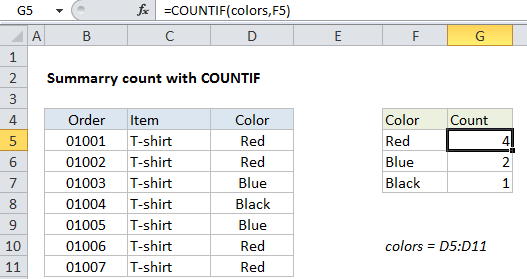## Excel Office

Excel How Tos, Tutorials, Tips & Tricks, Shortcuts

# Summary count with COUNTIF in Excel

This tutorial shows Summary count with COUNTIF in Excel using the example below;

## Formula

`=COUNTIF(range,criteria)`## Explanation

When working with data, a common need is to perform summary calculations that show total counts in different ways. For example, total counts by category, color, size, status, etc. The COUNTIF function is a good way to generate these kinds of totals.

### Example problem and solution

In the example shown, we have a small set of order data. All orders are for T-shirts, which come in 3 different colors: red, blue, and black.

Worked Example:   COUNTIFS with multiple criteria and OR logic in Excel

To the left, we are using COUNTIF to provide a breakdown by color. The formula in cell G5 is:

`=COUNTIF(colors,F5)`

where “colors” is a named range for cells D5:D11. We are using a named range in this case to make the formula easy to copy down the summary table. Alternately, you could use an absolute reference like so:

`=COUNTIF(\$D\$5:\$D\$11,F5) // absolute address option`

### How this formula works

The COUNTIF function takes two arguments: a range of cells to count, and the criteria to use for counting. In this case we want to count by color, so we have set up a small table that lists all colors in column F. This allows us to use the the color names in column F both for labels, and for the criteria that goes into COUNTIF as the second argument.

Worked Example:   Count cells that contain specific text in Excel

In this case, we are testing equality, so we don’t need to use any logical operators. We can simply enter the named range “color” for range, and a reference to the adjacent cell in column F for the criteria.

Worked Example:   Count cells that contain either x or y in Excel